Study Materials

NCERT Solutions for Class 11th Mathematics

Page 1 of 4

Chapter 10. Straight Lines

Exercise 10.1

Exercise 10.1

Q1. Draw a quadrilateral in the Cartesian plane, whose vertices are (– 4, 5), (0, 7), (5, – 5) and (– 4, –2). Also, find its area.Solution: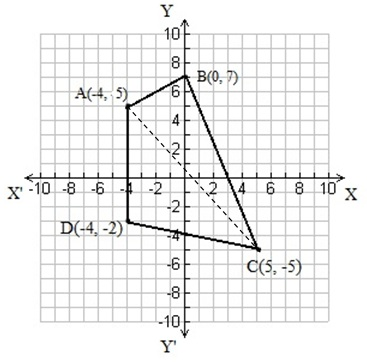Let ABCD be the given quadrilateral with vertices A (–4, 5), B (0, 7), C (5, –5), and D (– 4, –2).

Now, by plotting A, B, C, and D on the Cartesian plane and joining AB, BC, CD, and DA, the given quadrilateral can be drawn as joining Point A to C forms a diagonal AC.

Accordingly, area (ABCD) = area(∆ABC) + area (∆ACD)

Using area of triangle formula.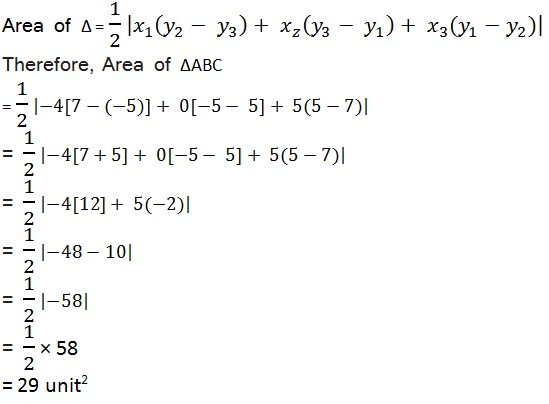Therefore, Area of ∆ACD where A (-4, 5), C (5, -5), D (-4, -2)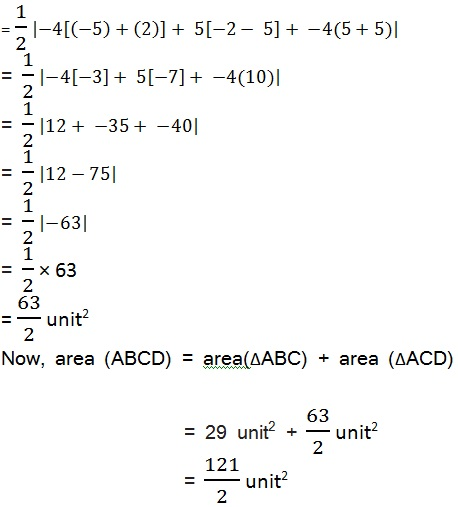Q2. The base of an equilateral triangle with side 2a lies along the y-axis such that the mid-point of the base is at the origin. Find vertices of the triangle.

Solution: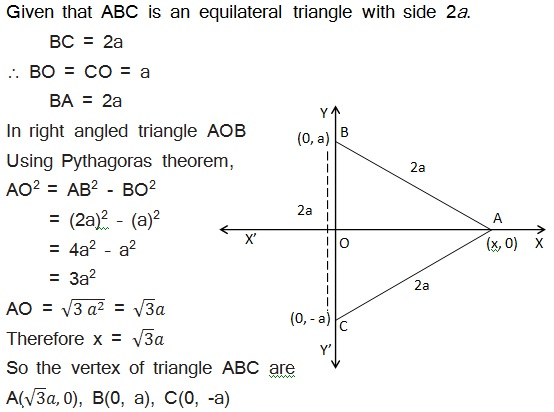Q3. Find the dis tance between P (x1, y1) and Q (x2, y2) when: (i) PQ is parallel to the y-axis, (ii) PQ is parallel to the x-axis.

Solution:

Given points are : P(x1, y1) and Q(x2, y2)

(i) When PQ is parallel to the y-axis then x1 = x2

Using Distance formula for distance between P and Q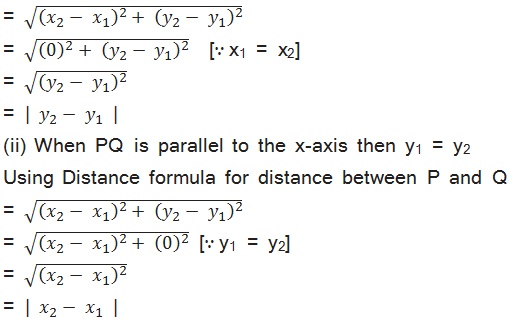Q4. Find a point on the x-axis, which is equidistant from the points (7, 6) and (3, 4).

Solution:

Let the point on x-axis be P (x, 0) which is equidistance from points A (7, 6) and B (3, 4).

Accordingly,

AP = BP

Or    AP2 = BP2  [Squaring both sides]

(x – 7)2 + (0 – 6)2 = (x – 3)2 + (0 – 4)2

x2 – 14x + 49 + 36 = x2 – 6x + 9 + 16

x2 – 14x + 85 = x2 – 6x + 25

85 – 25 = 14x – 6x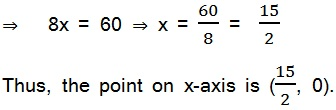Q5. Find the slope of a line, which passes through the origin and the mid-point of the line segment joining the points P (0, 4) and B (8, 0).

Solution:

The coordinates of the mid-point of the line segment joining the points P (0, 4) and B (8, 0).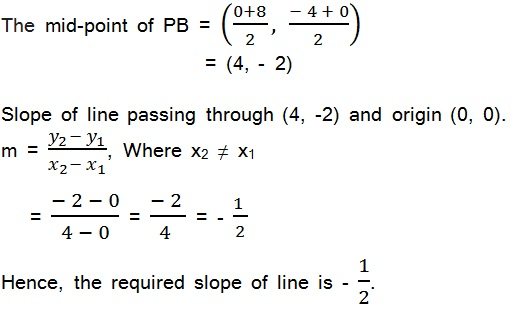Q6. Without using the Pythagoras theorem, show that the points (4, 4), (3, 5) and (1, 1) are the vertices of a right angled triangle.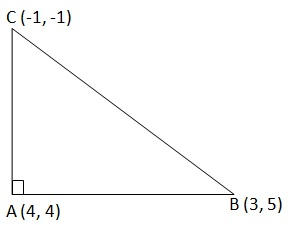Solution:

The vertices of the given triangle are

A (4, 4), B (3, 5), and C (–1, –1).

If given vertices are of a right angle triangle.m1m3 = -1

Slope of AB (m1) × Slope of AC (m3) = -1

It means side AB and AC are perpendicular to each other.

Here, given triangle is right-angled at point A (4, 4).

Thus, the points (4, 4), (3, 5), and (–1, –1) are the vertices of a right-angled triangle.

Q7. Find the slope of the line, which makes an angle of 30° with the positive direction of y-axis measured anticlockwise.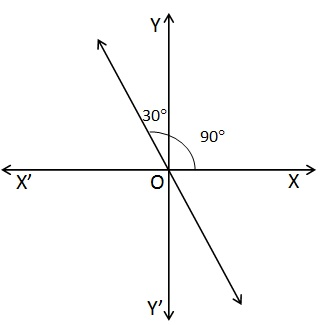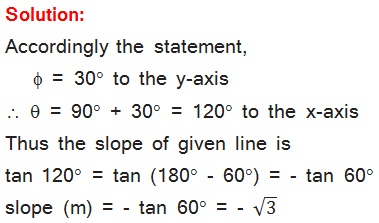Q8. Find the value of x for which the points (x, – 1), (2,1) and (4, 5) are collinear.

Solution:

Let point be A (x, –1), B (2, 1), and C (4, 5).

If points A (x, –1), B (2, 1), and C (4, 5) are collinear, then

Slope of AB = Slope of BC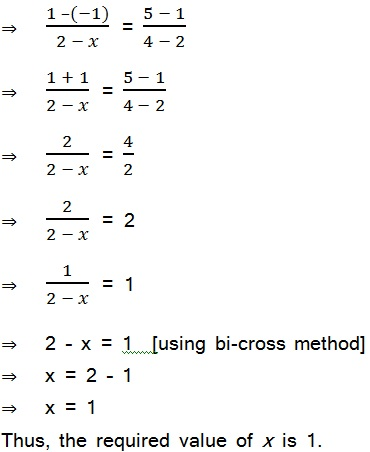Q9. Without using distance formula, show that points (2, 1), (4, 0), (3, 3) and (3, 2) are the vertices of a parallelogram.

Solution:

To be the points A(2, 1), B(4, 0), C(3, 3) and D(3, 2) of the vertices of a parallelogram.

There must be AB || CD or BC || AD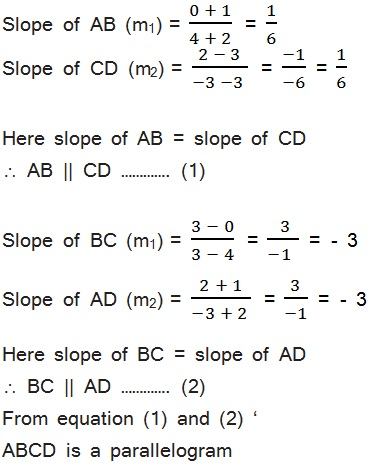Thus, points (–2, –1), (4, 0), (3, 3), and (–3, 2) are the vertices of a parallelogram.

Q10. Find the angle between the x-axis and the line joining the points (3,1) and (4,2).

Solution:

Let be points A(3,1) and B(4,2) are given for a line.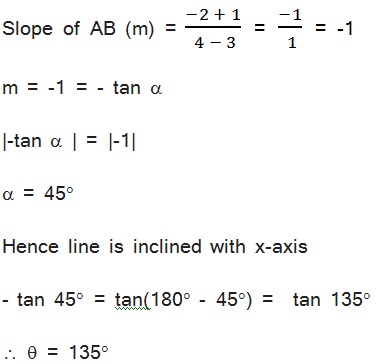Thus, the angle between the x-axis and the line joining the points (3, –1) and (4, –2) is 135°.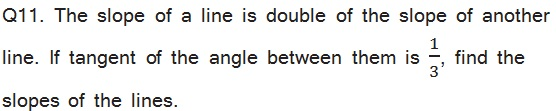Solution: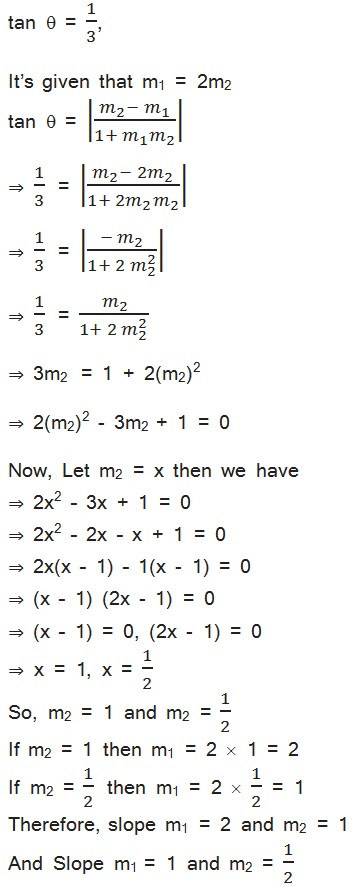Q12. A line passes through (x1, y1) and (h, k). If slope of the line is m, show that k – y1 = m (h – x1).

Solution:

Line passes through points (x1, y1) and (h, k).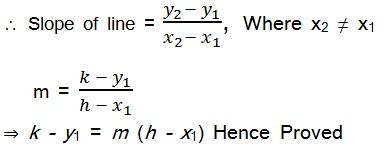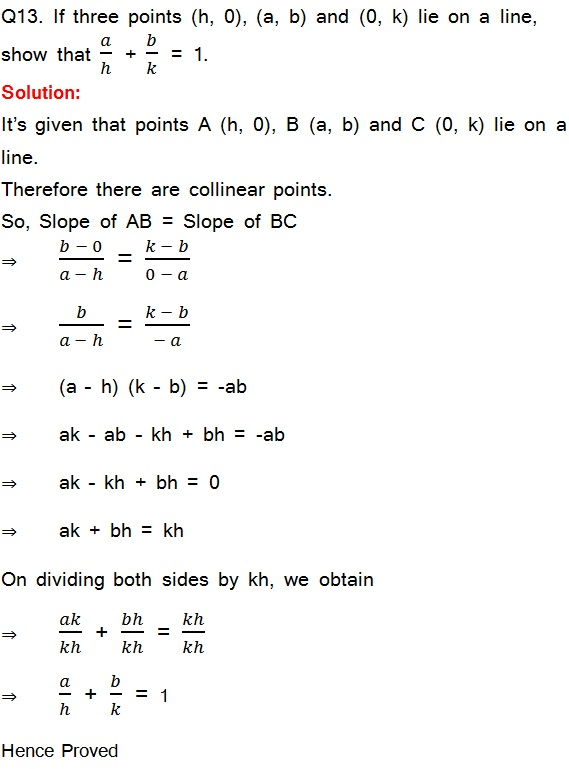Q14. Consider the following population and year graph, find the slope of the line AB and using it, find what will be the population in the year 2010?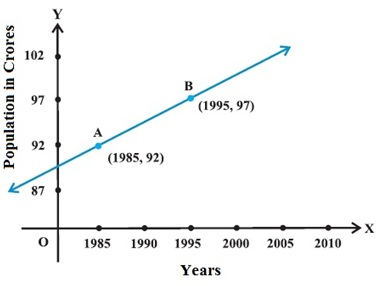Solution:

Line AB passes through points A(1985, 92) and B(1995, 97).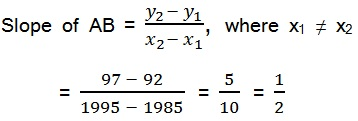Let y be the population in the year 2010. Then, according to the given graph, line AB must pass through point C (2010, y).Page 1 of 4

Chapter Contents: